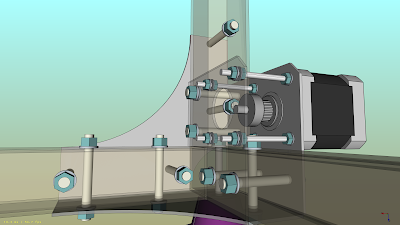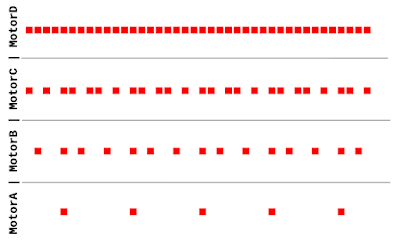## Thursday, 29 October 2015

### Arduino: Move 4 Stepper Motors Synchronously

...but, theoretically, it would work for an n number of motors.Part of the machine inside FreeCAD
This is the algorithm that plans the trajectory and syncs the movements of the stepper motors, in the new CNC machine that I'm designing (and building!)

The input is an int array { MA, MB, MC, MD } where each int means the number of steps (positive or negative).

The stepper movement logic is stored into a boolean array { SA, DA, SB, DB.... } where SA means step for motor A and DA is the direction bit of the motor A (for a 2 wire stepper driver, like the a4988). This array is erased and re-computed every loop cycle with the next movement, so real motor stepping has to take place inside this loop.

The image below (created with FreeCAD) shows a representation of the algorithm output for a requested movement of ( 5, 15, 25, 40 ) steps.Input ( 5, 15, 25, 40 ), step bit only

It would be possible to do some kind of buffer by translating that boolean array into an int inside an int array. This should be a more-less memory efficient way of decoupling motion logic and motion execution, something to try in a future.

The code:

```/*
* JMG October 2015
* NiCr Stepper Sync Algorithm V1
* Tested on Arduino Nano (Ide 1:635)
*
*/

// stepper_instruction { Step1A, Dir1A, Step1B, Dir1B, Step2A, Dir2A, Step2B, Dir2B }
bool stepper_instruction = { 0, 0, 0, 0, 0, 0, 0, 0 };

void moveAB( int Adx, int Ady, int Bdx, int Bdy )
{
int delta = { Adx, Ady, Bdx, Bdy };
int aux = 0;
for( int i = 0; i < 4; i++ )
{
if( abs(delta[i]) > aux )
{
aux = abs(delta[i]);
}
}
float R = { 0, 0, 0, 0 };
for( int i = 0; i < 4; i++ )
{
R[i] = (float)delta[i] / (float)aux;
}
int inc = { 0, 0, 0, 0 };
int acc = { 0, 0, 0, 0 };
int j = 0;
while( ( acc != Adx )||( acc != Ady )||( acc != Bdx )||( acc != Bdy ) )
{
j++;
for( int i = 0; i < 4; i++ )
{
inc[i] = round( R[i]*j - acc[i] );
acc[i] = acc[i] + inc[i];
stepper_instruction[2*i] = abs( inc[i] );
if( inc[i] < 0 ) { stepper_instruction[2*i+1] = 1; }
else { stepper_instruction[2*i+1] = 0; }
}
for( int i = 0; i < 7; i++ )
{
Serial.print( stepper_instruction[i] );
}
Serial.println( stepper_instruction );
for( int i = 0; i < 8; i++ )
{
stepper_instruction[i] = 0;
}
}
for( int i = 0; i < 4; i++ )
{
Serial.print( acc[i] );
}
Serial.println();
for( int i = 0; i < 4; i++ )
{
Serial.print( delta[i] );
}
}
void setup()
{
Serial.begin( 115200 );
delay( 500 );
moveAB( 100, -5000, 780, 25 );
}
void loop()
{
}
```

It prints by serial (115200 baud) the values of stepper_instruction at each loop cycle, and the counted steps vs the requested steps at the end of the loop.
I'm sure this is not the optimal way of doing it, but it works.

Update: the algorithm in action

PS:
I will reveal more info about the machine soon. NiCr